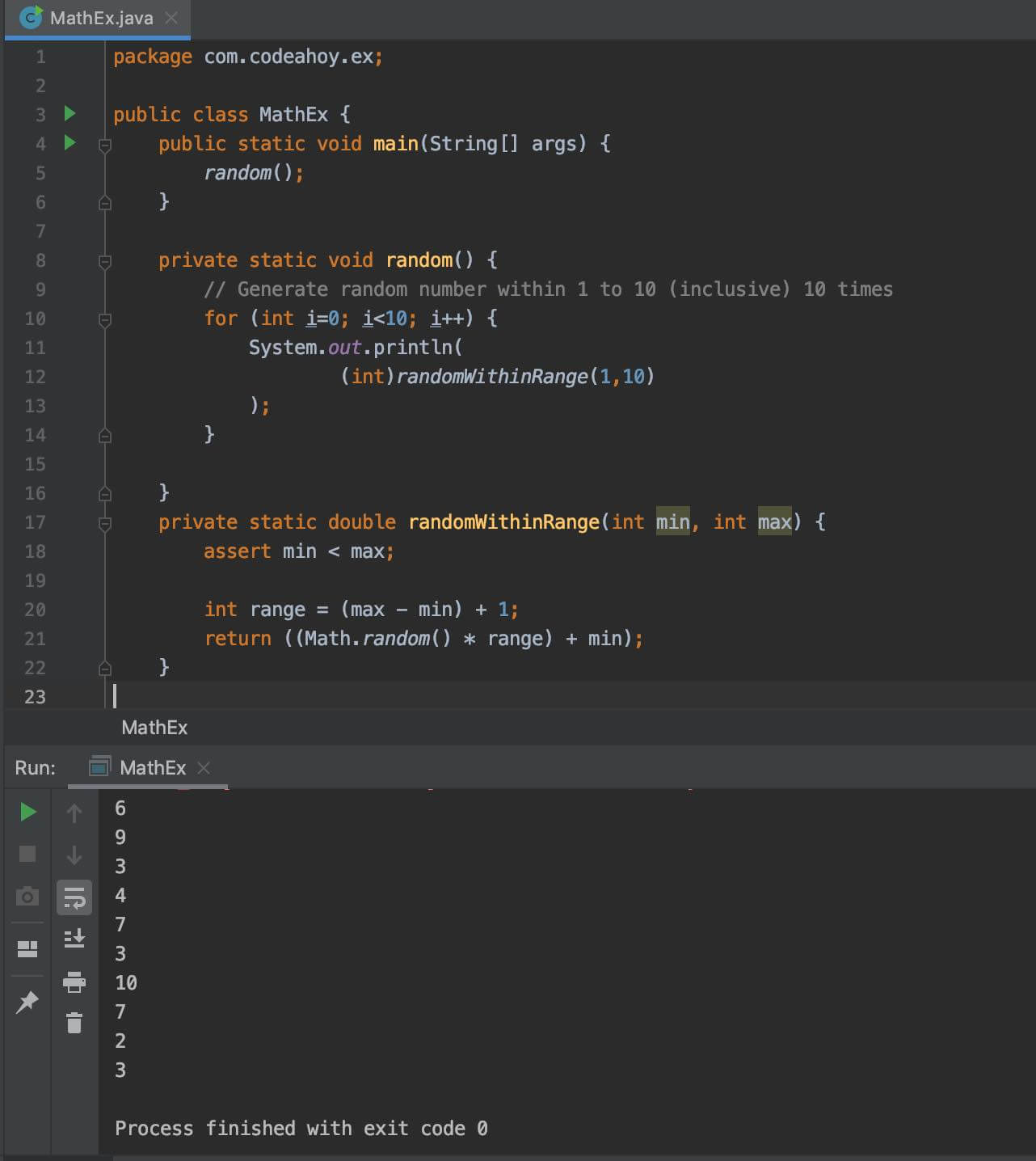# Java Math.random() Method with Examples

Oct 12, 2019 · 3 mins readThe java.lang.Math class that comes bundled with Java contains various methods for performing basic numeric operations such as the elementary exponential, logarithm, square root, and trigonometric functions.

Math.random() method is part of the Math class. It is used for generating random values between 0.0 and 0.1. The random values are chosen pseudorandomly. This means the algorithm relies solely on mathematical equations to generate random numbers. In contrast, true random number generators use unpredictable and external physical criteria (e.g. atmospheric data) to generate random numbers. If you’re looking for true random number generation in Java, take a look at javax.crypto.SecureRandom. It uses your system’s source for randomness (e.g. `/dev/urandom`) to generate random numbers. I digress, but if you’re interested in learning more about pseudorandom vs true random, here’s a good blog post by Bo Allen.

Let’s look at the method signature of the Math.random() method:

``````public static double random()
``````

Note that just like all other methods of the Math class, Math.random() is a `static` method so you can call it directly on the Math class without needing an object. It returns a value of type `double`. Another thing to keep in mind is that this method creates a new object of type Random which it uses internally to generate random numbers. The same random generator object is used for all calls to this method from anywhere in your code.

``````Math.random();  //  e.g. 0.5674616450812238
``````

## Example: Random Numbers Within a Range

A common use case is finding random numbers between a range. Let’s see an example where we’ll generate a random number within 1 to 10.

``````public class MathEx {
public static void main(String[] args) {
random();
}

private static void random() {
// Generate random number within 1 to 10 (inclusive) 10 times
for (int i=0; i<10; i++) {
System.out.println(
(int)randomWithinRange(1,10)
);
}

}
private static double randomWithinRange(int min, int max) {
assert min < max;

int range = (max - min) + 1;
return ((Math.random() * range) + min);
}
}
``````

Here’s output from the code above generating random number between 1 and 10 when run 10 times.

Output

``````6
9
3
4
7
3
10
7
2
3
``````

Here’s a screenshoot of the code above if you’re on mobile and having trouble reading the code above.### Special cases

• This method is properly synchronized to allow correct use by more than one thread. However, if many threads need to generate pseudorandom numbers at a great rate, it may reduce contention for each thread to have its own pseudorandom-number generator.

If you need more detailed information, please see Javadocs. It’s worth noting that the `Math` class in Java contains several other useful methods for arithmetic, logarithms and trignometric operations.

### Java Versions Tested

The examples in this post were compiled and executed using Java 8. Everything contained in this post is accurate to all versions up to Java 13 (which is the latest version.)

#### You May Also Enjoy

If you like this post, please share using the buttons above. It will help CodeAhoy grow and add new content. Thank you!